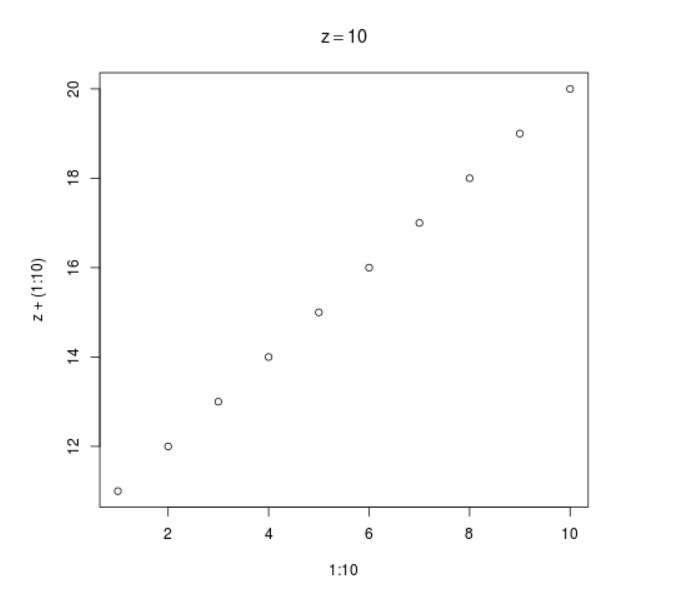Open In App

# Evaluate and Quote an Expression in R Programming – bquote() Function

`bquote()` function in R Language is used to quote the arguments passed to it, except the values which are wrapped in ‘.()‘. It evaluates the wrapped values and quotes the result.

Syntax: bquote(expr)

Parameters:
expr: language object

Example 1:

 `# R program to quote an expression`` ` `# Assigning value to variable``x <``-` `10`` ` `# Calling bquote() Function``bquote(x ``=``=` `x)``bquote(x ``=``=` `10``)``bquote(x ``=``=` `.(x))``bquote(x ``=``=` `.(x ``*` `2``))`

Output:

```x == x
x == 10
x == 10
x == 20
```

Example 2:

 `# R program to quote an expression`` ` `# Assigning value to variable``z <``-` `10`` ` `# Calling bquote() Function``bquote(function(x, y ``=` `.(z)) x ``+` `y)`` ` `# Plotting a graph with the default value``plot(``1``:``10``, z``+``(``1``:``10``), main ``=` `bquote(z ``=``=` `.(z)))`

Output:

```function(x, y = 10) x + y
```